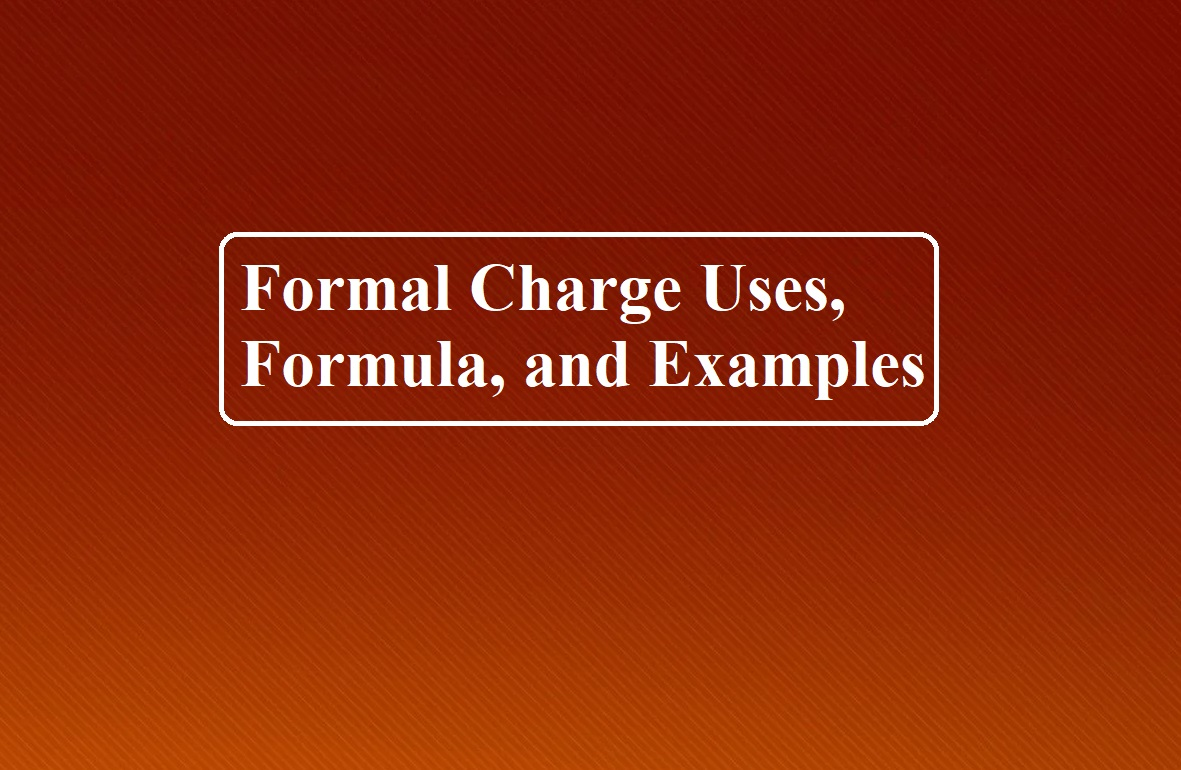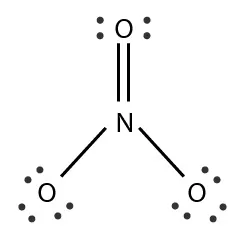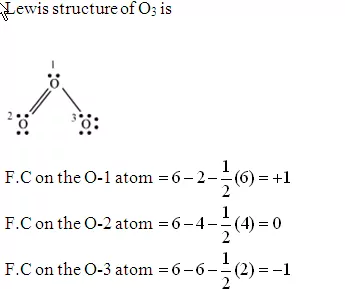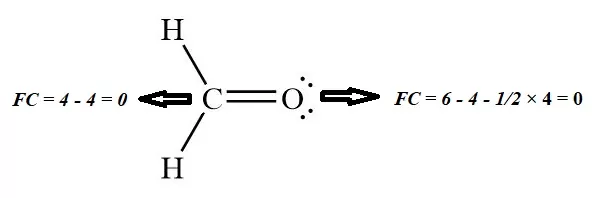# Formal Charge Uses, Formula, and Examples# Formal Charge Uses, Formula, and Examples

Formal Charge Uses, Formula, and Examples

Formal Charge: A formal charge (FC) is the charge assigned to an atom in a molecule.  The formal charge (F.C.) is the charge assigned to an atom in a molecule, assuming that the electrons in all chemical bonds are shared equally between atoms.
When determining the best Lewis Structure (or predominant resonance) for a molecule, the structure is chosen such that the formal charge on each of the atoms is as close to zero as possible.
In order to calculate a formal charge, electrons are assigned to individual atoms within the molecule based on two different rules: bonding electrons must be divided equally across the different bonded atoms, and non-bonding electrons are considered part of the atom they are located.
Formula to calculate Formal Charge:

FC = VE – NE – ½ BE

VEValence Electrons

NENon-Bonding Electrons

BEBonding Electrons

Uses of Formal Charge:
1. The formal charge being a theoretical charge doesn’t indicate any real charge separation in the molecule.
2. Formal charges help in the selection of the lowest energy structure from a number of possible Lewis structures for a given species.
3. Knowledge of the lowest energy structure helps in predicting the major product of a reaction and also describes a lot of phenomena.
4. Generally, the lowest energy structure is the one with the smallest formal charges on the atoms and the most distributed charge.

Examples to Calculate Formal charge:

1. Formal Charge on N in NO3 :Valence Electrons of N = 5,               Non-Bonding Electrons of N = 0

Bonding Electrons of N = 10

According to formula FC on N = 5 – 0 – ½ × 10 = 0

2. Formal charge on each oxygen in O3 Molecule:3. Formal charge on Cl atom of HClO4:FC = 7 – 0 ½ × 14 = 0

4. The formal charge on Carbon and oxygen of HCHO:

FC = VE – NE – 1/2 BEFormal Charge Uses, Formula, and Examples